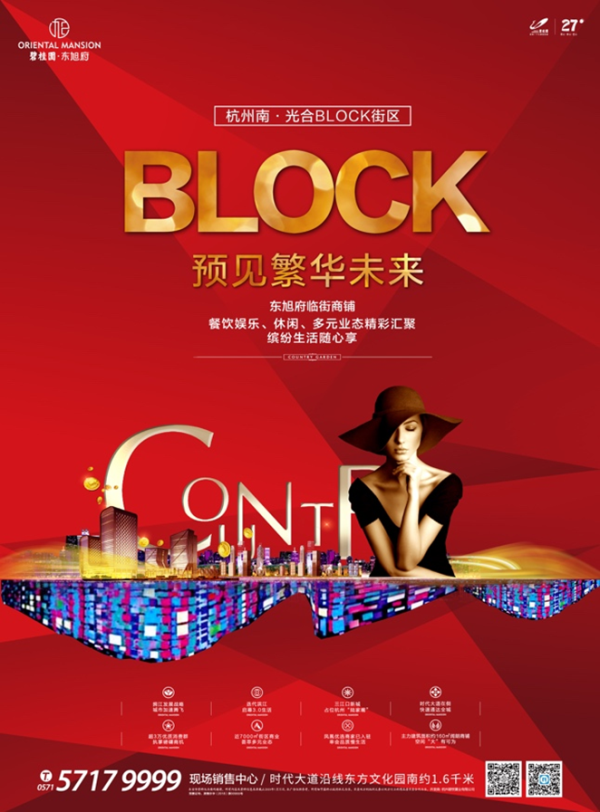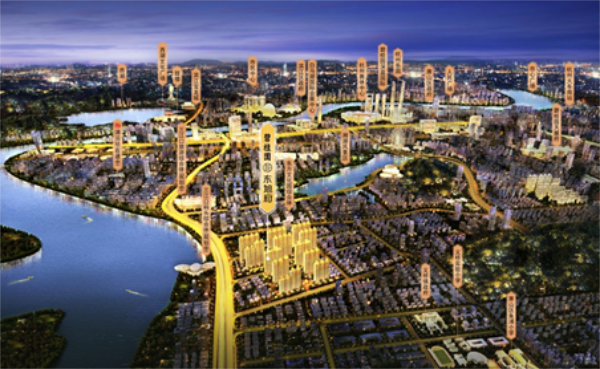## 网站地图## 碧桂园•东旭府

### 杭州

#### 地址

• 资阳
• 本溪
• 北流
• 万载
• 慈溪
• 长春
• 通化
• 西昌
• 丰城
• 阳春
• 鹤山
• 江油
• 辛集
• 辽源
• 衡水
• 黔西南州
• 黔东南州
• 石家庄
• 唐山
• 秦皇岛
• 邯郸
• 邢台
• 保定
• 张家口
• 承德
• 沧州
• 廊坊
• 黄骅
• 迁安
• 怀来
• 洛阳
• 驻马店
• 郑州
• 开封
• 平顶山
• 安阳
• 鹤壁
• 新乡
• 焦作
• 濮阳
• 许昌
• 漯河
• 三门峡
• 南阳
• 商丘
• 信阳
• 周口
• 武汉
• 黄石
• 十堰
• 宜昌
• 襄阳
• 荆州
• 荆门
• 鄂州
• 仙桃
• 潜江
• 黄冈
• 随州
• 咸宁
• 恩施
• 怀化
• 长沙
• 株洲
• 湘潭
• 湘西州
• 张家界
• 益阳
• 郴州
• 衡阳
• 岳阳
• 常德
• 娄底
• 徐州
• 南京
• 无锡
• 镇江
• 泰州
• 淮安
• 舟山
• 黔西南布依族苗族自治州
• 临沂
• 凉山
• 普洱
• 红河
• 锦州
• 葫芦岛
• 东方
• 咸阳
• 丽水
• 定安县
• 吕梁
• 来宾
• 河池
• 宜宾
• 重庆市
• 雅安
• 潮州
• 大理
• 巴中
• 桂林
• 贵港
• 百色
• 柳州
• 贺州
• 儋州
• 福清
• 威海
• 烟台
• 乐山
• 金华
• 永泰
• 潍坊
• 滨州
• 德州
• 临汾
• 日照
• 眉山
• 武威
• 乌鲁木齐
• 保山
• 临沧
• 防城港
• 达州
• 遂宁
• 绵阳
• 淮南
• 宝鸡
• 枣庄
• 桂平
• 景德镇
• 临夏
• 聊城
• 崇左
• 马鞍山
• 安顺
• 北海
• 菏泽
• 铜陵
• 黔南州
• 蚌埠
• 嘉兴
• 淮北
• 都匀
• 南宁
• 兴义
• 南平
• 松溪
• 吉安
• 大同
• 陆丰
• 厦门
• 铜仁
• 银川
• 昭通
• 包头
• 遵化
• 漳州
• 深圳
• 霸州
• 香港
• 合肥
• 抚州
• 琼海
• 定西
• 南昌
• 宿州
• 文昌
• 临高县
• 陵水
• 海口
• 博鳌
• 三亚
• 绥化
• 哈尔滨
• 杭州
• 绍兴
• 宁波
• 温州
• 衢州
• 湖州
• 台州
• 天津
• 沈阳
• 大连
• 通辽
• 兴安盟
• 呼伦贝尔
• 运城
• 晋中
• 太原
• 南通
• 宿迁
• 扬州
• 盐城
• 连云港
• 苏州
• 常州
• 安庆
• 黄山
• 巢湖
• 芜湖
• 池州
• 宣城
• 六安
• 古田
• 无为
• 桐城
• 亳州
• 南陵
• 庐江
• 阜阳
• 滁州
• 孝感
• 天门
• 邵阳
• 永州
• 平果
• 玉林
• 梧州
• 钦州
• 梅州
• 河源
• 云浮
• 东莞
• 汕头
• 中山
• 清远
• 惠州
• 韶关
• 肇庆
• 阳江
• 汕尾
• 茂名
• 江门
• 佛山
• 广州
• 雷州
• 英德
• 湛江
• 揭阳
• 珠海
• 信宜
• 济南
• 淄博
• 青岛
• 泰安
• 济宁
• 马来西亚雪兰莪州
• 马来西亚柔佛州
• 永春
• 南安
• 三明
• 宁德
• 武夷山
• 福鼎
• 莆田
• 建瓯
• 漳浦
• 福安
• 福州
• 泉州
• 龙岩
• 兰州
• 张掖
• 天水
• 白银
• 贵阳
• 仁怀
• 毕节
• 凯里
• 六盘水
• 遵义
• 广元
• 德阳
• 南充
• 泸州
• 攀枝花
• 广安
• 成都
• 宜春
• 萍乡
• 九江
• 赣州
• 上饶
• 曲靖
• 楚雄
• 昆明
• 白城
• 北京
• 澳洲莱德
• 西安
• 渭南
• 上海
• 西宁雄踞杭州三江口 傲揽璀璨前景

伴随“拥江发展”战略推进，三江口新城的打造备受瞩目。地铁、商业、医疗等配套陆续规划建设，生态和生活资源兼备，板块未来可期。碧桂园·东旭府光合BLOCK街区雄踞杭州三江口，更将以新兴商业形态驱动板块整体商业格局稳步成熟。

精工布局 光合BLOCK街区

碧桂园·东旭府借鉴国外街区生态链进行整体规划，打造适合多元业态经营的光合BLOCK街区。近7000㎡体量，建筑面积约40-283㎡商铺空间，1+2建筑结构，超5米大气开间布局，更以科学进深和大尺度层高提升空间功能性，保障商铺高效运营。多元业态规划 优享体验式商业

43间街铺涵盖餐饮、品牌超市等多元业态，不出小区即可享受丰盛生活。目前，凤凰优选品牌店已入驻碧桂园·东旭府南向街铺，北区7、8号楼则规划为餐饮业态。集餐饮、休闲、娱乐、购物于一体的BLOCK街区，将为您带来体验式商业生活。

消费人气爆棚 未来商机无限

碧桂园·东旭府光合BLOCK街区人气汇聚。除东旭府1500多户业主之外，周边更有金域兰庭1400多户业主，御景蓝湾5900多户业主，江湾城2000多户业主。一席街区商铺，执掌未来商机。

凤凰优选品牌店 强势入驻

凤凰优选以社区零售为基础，包含大中小型社区店、无人店、自动贩售机、在线商城等业态组合，同时具备凤凰优选app、凤凰优选无人超市小程序等线上应用。引入互联网概念，消费者扫码购物、自主结算，新零售元素丰富。选品则考察全球原产地，从源头把控食品质量，为您带来安全、健康、优质的生活品质。

悠闲生活场域 城市难得慢时光

快节奏城市生活逐渐模糊了生活本质。东旭府BLOCK街区，为年轻人带来身心放松的舒适所在。无论是滨江上班族，还是萧山本地白领，东旭府BLOCK街区将承载你的闲暇时刻。在结束一天的忙碌之后，尽享难得慢时光。品牌见证实力 成就智慧之选

碧桂园集团，世界500强，中国地产三强。立业20余载，已为超过1100个城镇带来现代化的城市面貌，现已打造超过2000个高品质项目，有超400万业主选择在碧桂园安居乐业。碧桂园·东旭府光合BLOCK商业街区，将在集团雄厚品牌实力积淀下，成就你的智慧之选。